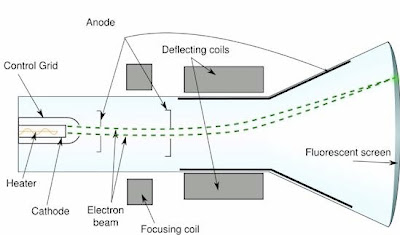## July 17, 2012

### CRT Oscilloscope, Function Generator & Frequency Calculation

This is the 10th and last experiment of our 1st semester Applied Physics lab course. Now, we are going to discuss that how to to use CRT Oscilloscope and Function generator to calculate Frequency, Wavelength and Time period.

The purpose of sharing this topic is to help the fellow and new coming students for preparation of their Lab Manuals. I performed this experiment in university physics lab.

Keep reading for circuit diagrams, block diagrams and full range of all the value tables.

Experiment # 10

Use of CRT Oscilloscope & Function Generator to calculate Frequency

Apparatus: Multi-meter, Oscilloscope, Function Generator, AC Power Supply.

What is Oscilloscope ?

An Oscilloscope is an electronic device which can display and measure many electric quantities. It can measure:
• Ac /Dc voltages
• Frequency
• Time Period
• Phase difference and much more..."CRT Oscilloscope"
Function Generator:

We can get different types of wave shapes from a function generator. A function generator has a frequency range and we can get varying amplitude from its main output. We can verify its frequency and wave shapes by applying its output to oscilloscope.

For using a function generator we plug its probe in any of the output jack 20V p-p or 15V p-p. Select the required frequency and amplitude from there select switches. Moreover, different wave shapes are available. The wave shape can be observed on the oscilloscope and can be easily adjusted.Cathode Ray Tube
Procedure for Voltage Measurement:
1. Connect the signal to be measured to the input jack and select CH1/CH@ to be used.
2. Set the volts/Div and time/Div controls to obtain a wave form.
3. Adjust the reference level of the trace by POS control. Make sure to calibrate the oscilloscope.
4. Set the AC-GND-DC switch to DC or AC.
5. Use the x POS and Volts/Div control to set the waveform.
6. Multiply the vertical measured distance (no. of large divisions on oscilloscope) with volts/Div and probe magnification.
7. Similarly V p-p can be measured for AC voltages by counting the no. of division.
Observations for Voltage:

Procedure for Frequency & Time Period Measurement:
1. Connect the signal to be measured to CH1 / CH2. Also switch the channel.
2. Set the volts per division knod and time per division knob to obtain a normal waveform.
3. Keep the VAR SWEEP control to CAL.
4. Using POS control, adjust the references at the centre of horizontal and vertical line.
5. Measure the Horizontal distance between two points. Multiply this by the Time/Div control and magnification: Time = Horizontal divisions X Time / Div (X (1/10) if X10 is used)
6. Frequency measurements are made by measuring the time taken by one cycle, Frequency1/Time period.
Observations for F & T :

This is all the steps to measure frequency using Cathode Ray Tube Oscilloscope.